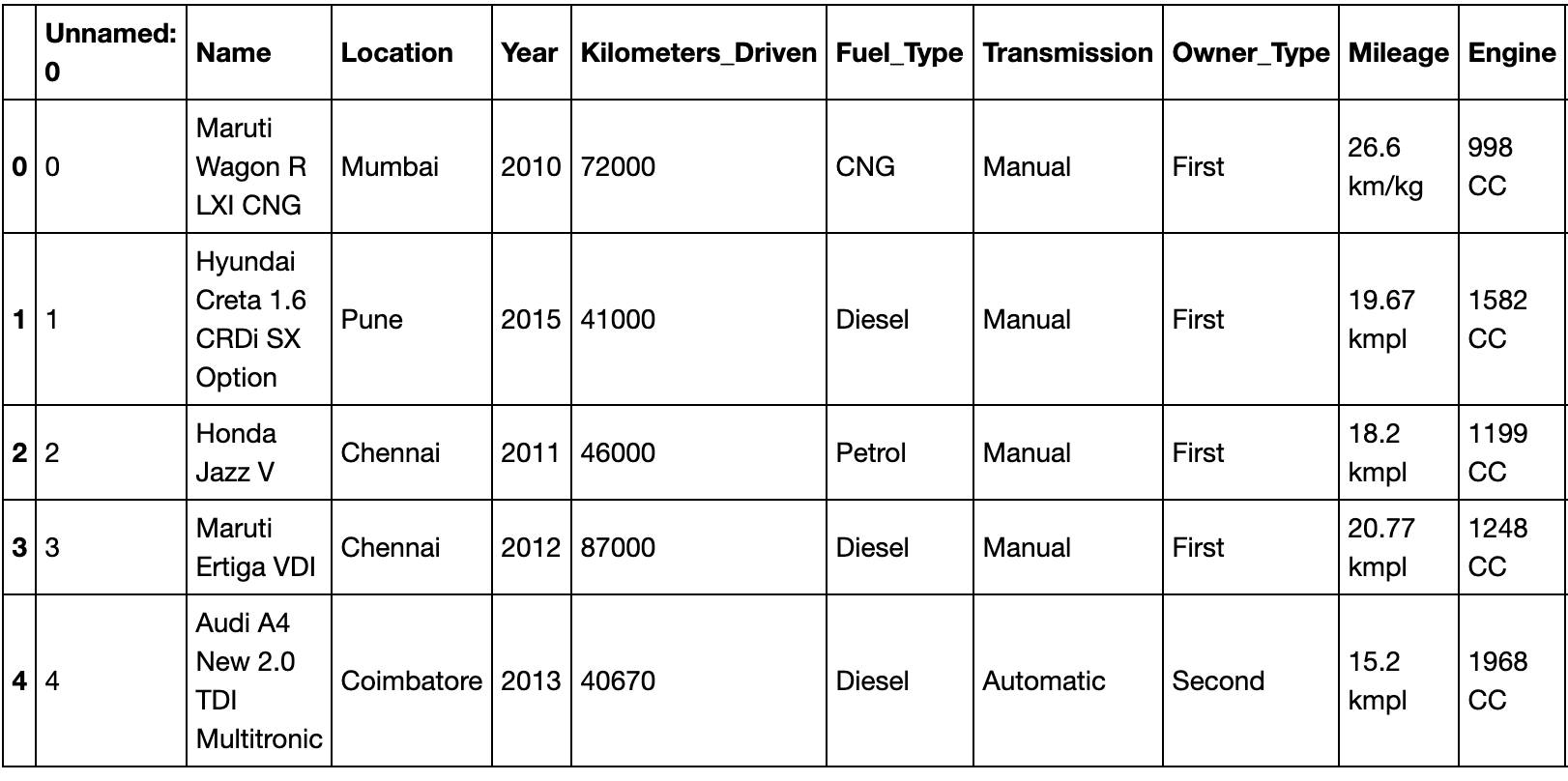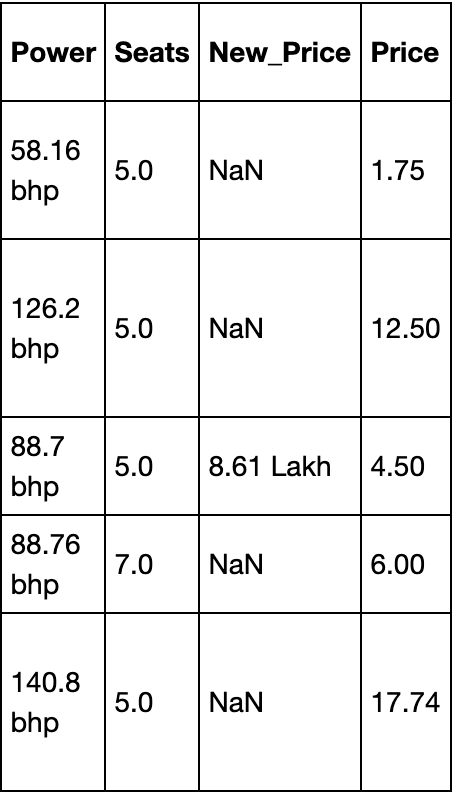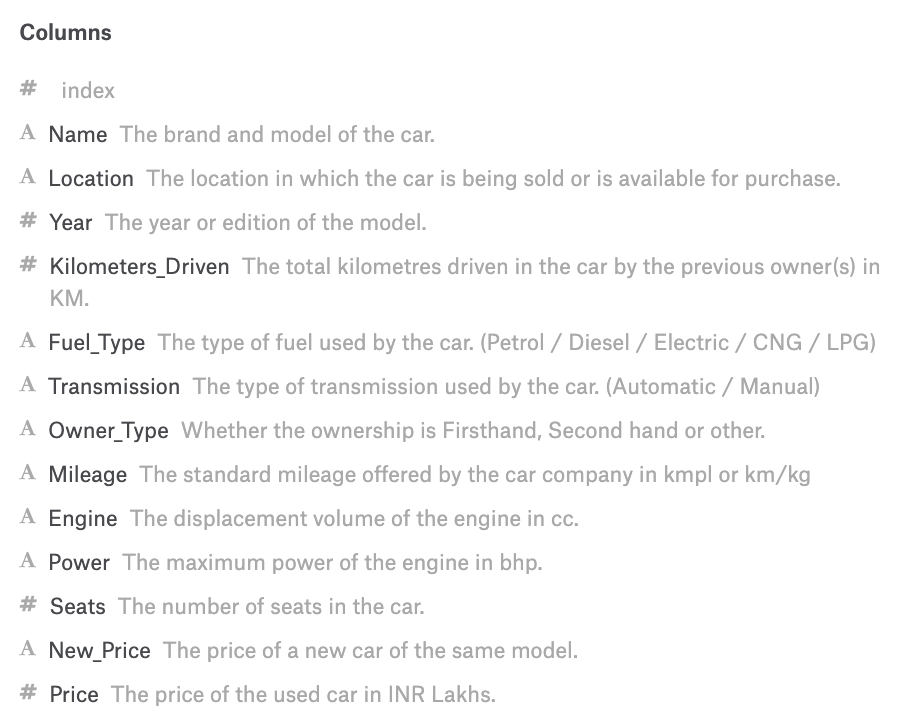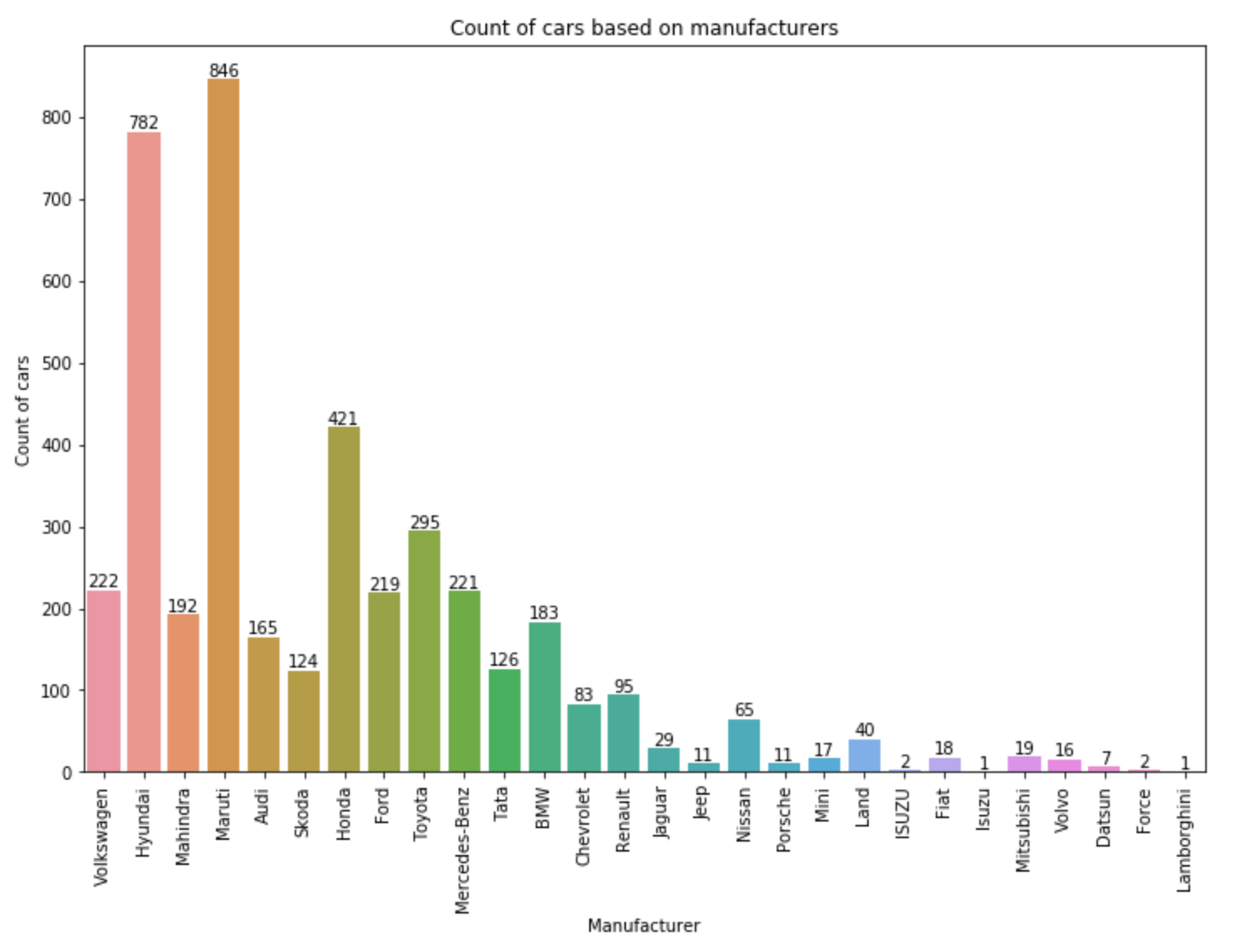# Improve your ML model by trying different approaches

Used cars price prediction using Machine LearningPhoto by Jen Theodore on Unsplash

In this article, we’ll take a look at the recent project I completed where I predicted the prices of used cars based on a number of factors. I found the dataset on Kaggle.

This project is special as I tried many different things and then finalized on the notebook that is included as part of the repository. I’ll explain each and every step I thought and how it turned out to be. The repository with the code is below:

Here’s the crux of the article:
1. Creating new features could be helpful e.g. I created the feature Manufacturer from Name.
2. Try different approaches to handle the same column. The Year column when used directly produced bad results so I instead used the age of each car derived from it which was much more useful. ``New_Price`` was first filled with average values based on ``Manufacturer`` but it was not useful, so I dropped the column in the second iteration.
3. Columns that seem irrelevant should be dropped. I dropped ``Index, Location, Name`` and ``New_Price`` .
4. Creating dummies requires handling of missing columns in test data.
5. Play around with the parameters of the ML model as it can be useful. The parameter ``n_estimators`` in RandomForestRegressor improved the ``r2_score`` when I set the value to 100. I also tried 1000 but it just took a lot longer without any noticeable improvement.

If you still want the complete details, keep reading!

Importar Librerias
I’ll import ``datetime`` library to work with the ``Year`` column. The ``numpy`` and ``pandas`` libraries help me work with the dataset. ``matplotlib`` and ``seaborn`` help in plotting which I didn’t do much in this project. Finally, I import a number of things from ``sklearn`` especially metrics and models.

```import datetime

import numpy as np
import pandas as pd

import matplotlib.pyplot as plt
import seaborn as sns
%matplotlib inline

from sklearn.model_selection import train_test_split
from sklearn.linear_model import LinearRegression
from sklearn.ensemble import RandomForestRegressor
from sklearn.preprocessing import StandardScaler
from sklearn.metrics import r2_score```

The original Kaggle dataset had two files: ``train-data.csv`` and ``test-data.csv`` . However, the final output labels for the file ``test-data.csv`` were not given and hence, I would never be able to test my model. Thus, I decided to just work with the ``train-data.csv`` and rename it as ``dataset.csv`` inside the ``data`` folder.

```dataset = pd.read_csv("data/dataset.csv")dataset.csv (Part 1)dataset.csv (Part 2)

I then split the dataset into 70% training and 30% testing data.

```X_train, X_test, y_train, y_test = train_test_split(dataset.iloc[:, :-1],
dataset.iloc[:, -1],
test_size = 0.3,
random_state = 42)

X_train.info()

## Output
# <class 'pandas.core.frame.DataFrame'>
# Int64Index: 4213 entries, 4201 to 860
# Data columns (total 13 columns):
# Unnamed: 0           4213 non-null int64
# Name                 4213 non-null object
# Location             4213 non-null object
# Year                 4213 non-null int64
# Kilometers_Driven    4213 non-null int64
# Fuel_Type            4213 non-null object
# Transmission         4213 non-null object
# Owner_Type           4213 non-null object
# Mileage              4212 non-null object
# Engine               4189 non-null object
# Power                4189 non-null object
# Seats                4185 non-null float64
# New_Price            580 non-null object
# dtypes: float64(1), int64(3), object(9)
# memory usage: 460.8+ KB```

I output the training data information to see what the data looks like. We find that some columns like ``Mileage, Engine, Power`` and Seats have a few null values while ``New_Price`` has majority of its values missing. To get a better essence of what each column really represents, we can take a look at the Kaggle dashboard that has the data description.Columns description

The dataset is now loaded and we know what each column means. It’s now time to do some exploratory analysis. Note that I will always work with training part and then transform the test part based on the training part only.

Exploratory Data Analysis
Here, we’ll explore each of the columns above and discuss their relevance.

Index
The first column in the dataset is unnamed. It is actually just an index for each row and thus, we can safely remove this column.

```X_train = X_train.iloc[:, 1:]
X_test = X_test.iloc[:, 1:]```

Name
The ``Name`` column defines the name of each car. I thought the car name might not have a huge impact but the manufacturer of the car can. For example, if generally people find ``Maruti`` to produce reliable cars, their resale values should be higher. Thus, I decided to extract the ``Manufacturer`` from each ``Name`` . The first word of each ``Name`` is the manufacturer.

```make_train = X_train["Name"].str.split(" ", expand = True)
make_test = X_test["Name"].str.split(" ", expand = True)

X_train["Manufacturer"] = make_train
X_test["Manufacturer"] = make_test```
Let’s also plot and see the count of each cars based on the manufacturer.

```plt.figure(figsize = (12, 8))
plot = sns.countplot(x = 'Manufacturer', data = X_train)
plt.xticks(rotation = 90)
for p in plot.patches:
plot.annotate(p.get_height(),
(p.get_x() + p.get_width() / 2.0,
p.get_height()),
ha = 'center',
va = 'center',
xytext = (0, 5),
textcoords = 'offset points')

plt.title("Count of cars based on manufacturers")
plt.xlabel("Manufacturer")
plt.ylabel("Count of cars")```Manufacturer plot

As we can see in the plot above, Maruti has the maximum number of cars and Lamborghini has the least number of cars in the whole training data. Also, I don’t need the Name column so I dropped it.

```X_train.drop("Name", axis = 1, inplace = True)
X_test.drop("Name", axis = 1, inplace = True)```

Location
I initially tried to use ``Location`` but it lead to many one hot columns without contributing much towards the prediction help. This means that location of selling has almost negligible effect on the final resale price of a car. Thus, I decided to drop this column.

```X_train.drop("Location", axis = 1, inplace = True)
X_test.drop("Location", axis = 1, inplace = True)```

Year
I initially kept ``Year`` as it is to define the make of the model. But later did I realize that rather than the year, it’s how old the car is that has an effect on the resale value. Thus, taking cues from Kaggle, I decided to replace ``Year`` with the age of the car by subtracting the year from current year.

```curr_time = datetime.datetime.now()
X_train['Year'] = X_train['Year'].apply(lambda x : curr_time.year - x)
X_test['Year'] = X_test['Year'].apply(lambda x : curr_time.year - x)```

Fuel_Type, Transmission, and Owner_Type
All these columns are categorical columns. Thus, I’ll create dummy columns for each of these columns and use it for prediction.

Kilometers_Driven

```X_train["Kilometers_Driven"]

## Output
# 4201     77000
# 4383     19947
# 1779     70963
# 4020    115195
# 3248     58752
#          ...
# 3772     27000
# 5191      9000
# 5226    140000
# 5390     76414
# 860      98000
# Name: Kilometers_Driven, Length: 4213, dtype: int64```
The data output shows the high values that exist in the column. We should scale the data as well else columns like ``Kilometers_Driven`` can have a much stronger effect on prediction than other columns.

Mileage
``
Mileage`` defines the mileage of the car. However, the mileage units vary based on the type of engine e.g. some are per Kg while some are per L. But for this case, we’ll consider them equivalent and just extract the numbers from this column.

```mileage_train = X_train["Mileage"].str.split(" ", expand = True)
mileage_test = X_test["Mileage"].str.split(" ", expand = True)

X_train["Mileage"] = pd.to_numeric(mileage_train, errors = 'coerce')
X_test["Mileage"] = pd.to_numeric(mileage_test, errors = 'coerce')```
As we checked before, the Mileage column had some missing values so let’s check them and update the null values with the mean of the column.

```print(sum(X_train["Mileage"].isnull()))
print(sum(X_test["Mileage"].isnull()))

## Output
# 1
# 1

X_train["Mileage"].fillna(X_train["Mileage"].astype("float64").mean(), inplace = True)
X_test["Mileage"].fillna(X_train["Mileage"].astype("float64").mean(), inplace = True)```
Engine, Power y Seats
The ``Engine`` values are defined in CC so I need to remove CC from the data. Similarly, ``Power`` has bhp, so I’ll remove bhp from it. Also, as there are missing values in all three, I’ll again replace them with the mean as I did for ``Mileage`` .

I use ``pd.to_numeric()`` as handles null values and does not produce errors when converting from string to numerical (int or float).

```cc_train = X_train["Engine"].str.split(" ", expand = True)
cc_test = X_test["Engine"].str.split(" ", expand = True)
X_train["Engine"] = pd.to_numeric(cc_train, errors = 'coerce')
X_test["Engine"] = pd.to_numeric(cc_test, errors = 'coerce')

bhp_train = X_train["Power"].str.split(" ", expand = True)
bhp_test = X_test["Power"].str.split(" ", expand = True)
X_train["Power"] = pd.to_numeric(bhp_train, errors = 'coerce')
X_test["Power"] = pd.to_numeric(bhp_test, errors = 'coerce')

X_train["Engine"].fillna(X_train["Engine"].astype("float64").mean(), inplace = True)
X_test["Engine"].fillna(X_train["Engine"].astype("float64").mean(), inplace = True)

X_train["Power"].fillna(X_train["Power"].astype("float64").mean(), inplace = True)
X_test["Power"].fillna(X_train["Power"].astype("float64").mean(), inplace = True)

X_train["Seats"].fillna(X_train["Seats"].astype("float64").mean(), inplace = True)
X_test["Seats"].fillna(X_train["Seats"].astype("float64").mean(), inplace = True)```

New_Price
Most of the values in the column are missing. I initially decided to fill them up. I would fill the mean value based on the manufacturer. For example, for Ford, I’d take all values that are present, take their mean and then replace all null values of New_Price for Ford with that mean. However, this still left out a few null values. I would then fill these null values with mean of all the values in the column. The same was repeated for test data as well.

However, this approach wasn’t really successful. I tried to run the Random Forest Regressor on it and the results were very small ``r2_score`` values. Next, I decided that I would simply drop the column and the ``r2_score`` values improved significantly.

```X_train.drop(["New_Price"], axis = 1, inplace = True)
X_test.drop(["New_Price"], axis = 1, inplace = True)```

Data Processing
Here, I’ll create dummy columns using pd.get_dummies for all categorical variables.

```X_train = pd.get_dummies(X_train,
columns = ["Manufacturer", "Fuel_Type", "Transmission", "Owner_Type"],
drop_first = True)

X_test = pd.get_dummies(X_test,
columns = ["Manufacturer", "Fuel_Type", "Transmission", "Owner_Type"],
drop_first = True)```
However, it is quite possible that due to lack of all types in test data, there may be missing columns. Let’s understand it with an example. For example in the column ``Transmission`` , the training data includes ``Manual`` and ``Automatic`` so the dummies would be like ``Transmission_Manual`` and ``Transmission_Automatic`` . But what if the test data has only ``Manual`` value and no ``Automatic`` one. In such a case, dummies would only lead to ``Transmission_Manual`` . This will leave the test dataset one column short in comparison to training data and the prediction won’t work. To handle this, we create columns in test data that are missing and fill them with zero. Finally, we order the test data just like train data.

```missing_cols = set(X_train.columns) - set(X_test.columns)
for col in missing_cols:
X_test[col] = 0
X_test = X_test[X_train.columns]```

Lastly, I’d scale the data.

```linearRegression = LinearRegression()
linearRegression.fit(X_train, y_train)
y_pred = linearRegression.predict(X_test)
r2_score(y_test, y_pred)

rf = RandomForestRegressor(n_estimators = 100)
rf.fit(X_train, y_train)
y_pred = rf.predict(X_test)
r2_score(y_test, y_pred)```

Training and predicting
I’ll create a Linear Regression and a Random Forest model to train on the data and compare the ``r2_score`` values to select the best pick.

```linearRegression = LinearRegression()
linearRegression.fit(X_train, y_train)
y_pred = linearRegression.predict(X_test)
r2_score(y_test, y_pred)

rf = RandomForestRegressor(n_estimators = 100)
rf.fit(X_train, y_train)
y_pred = rf.predict(X_test)
r2_score(y_test, y_pred)```
I get the ``r2_score`` for Linear Regression as 0.70 and for Random Forest as 0.88. Thus, Random Forest performed really well on the test data.

Conclusion
In this article, we saw how to approach a machine learning problem in real life and how we might tweak features based on their relevance and the information they give out.

“Improve your ML model by trying different approaches”
– Karan Bhanot

### Related Stories

Jan 06, 2023

#### Top 2 Online Data Science Courses to Improve your Career in 2023

The discipline of Data Science is expanding quickly and has enormous promise. It is used in various sectors, including manufacturing, retail, healt...By Nikos_DataSource
Nov 25, 2021

#### 5 Tips To Ace Your Job Interview For A Data Scientist Opening

5 Tips To Ace Your Job Interview For A Data Scientist Opening.PNG 795.94 KBImage SourceAspiring data scientists have a bright future ahead of them....By Daniel Morales
Nov 12, 2021

#### When to Avoid Deep Learning